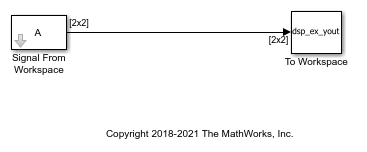# Import 3-D Array From Workspace

Import a 3-D array `A` from the MATLAB® workspace into the Simulink® model using the Signal From Workspace block. Use the To Workspace block to write the imported data to the MATLAB workspace. In this model, the To Workspace block slightly modifies data.

The parameters in the Signal From Workspace block are configured as follows:

• Sample time set to `1`: This parameter determines, Ts , the sample period of the output.

• Samples per frame set to `1`: Number of samples, Mo , to buffer into each output frame. The output frame period is MoTs.

• Form output after final data value by set to `Setting to zero`: The block generates zero-valued outputs for the duration of the simulation after generating the last frame of the signal.

Open and simulate the model.The Signal From Workspace block imports the signal from the variable `A`, which is a 2-by-2-by-3 array. When you simulate the model, each of the three pages (a 2-by-2 matrix) is output in sequence with period Ts. The third page is a matrix of zeros because the Form output after final data value by parameter is set to `Setting to zero` in this model. The To Workspace block writes the sequence of these matrices to the MATLAB workspace in the variable `dsp_ex_yout`.

This figure shows the signal in the input variable `A` and how this data is written to the output array `dsp_ex_yout`.